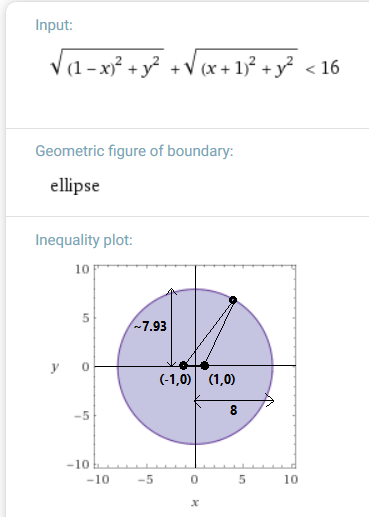## DATA-DRIVEN

Migrations are computed using an algorithm on a set of data. The data consists of a phylogenetic tree and geospatial samples on this tree, which may have birthdates.

Presently there is no fixing of clades to positions based on expert opinion.

As such it is a completely data-driven process.

## DATA

1. Download the YFull tree. This contains the hierarchy of male lineages:
• SNPs in each branching point
• estimates for start and end dates of the bottlenecks at each branching point
• ancient samples (how many) devoid of sample age and location code
• modern samples devoid of sample age but containing paternal ancestry location code
2. For ancient samples, use locations and sample ages from Ancient DNA public collaboration resource
3. Convert paternal ancestry location codes to latitude longitude using this script (link)
4. Exclude samples from the New World and Australia except for certain haplogroups (New World - C-1373, Q-Z780, Q-M3, Q-F746; Australia - A0-T, C, D, H, M, O, Q, R2, S, F-Y27277, K-Y28299)

## ALGORITHM v3.0 June 14, 2019

1. Remove outliers from samples of leaf nodes containing at least three samples. A sample is an "outlier" if:
• Its mean distance to the others is over 1000km
• For a group of three total points, its mean distance to others is over four times the distance of the other points from one another removalAny point outside the ellipse above (roughly a circle centered on the origin with radius 8) will be considered an outlier given two other points (-1,0) and (1,0). Solution and graph generated by Wolfram Alpha from formula above.
• For a group of four or more points, its mean distance to the others is over three times the distance of the other points from one another
2. Recursively calculate an initial average position for each branch
• For leaf nodes, average the samples
• For non-leaf nodes, include basal samples along with previously computed child average positions in the averaging process
• Averaging process applies a weight which is the reciprocal of the maximum of 500 and:
• (Sample) Elapsed time (years) between node's TMRCA and sample's birth
• (Branch node) Elapsed time (years) between node's TMRCA and child branch's TMRCA
3. Recursively refine the position of each clade by taking relative position of parent's initially computed average position to the refined children node positions/samples into account.
• Remove outliers from the child branch and basal sample positions as in 1.
• Point To Line refinement (2 points). Given P = parent, A = closest point, B = furthest point, M = midpoint:
• If PM < PA and PM < PB, use M
• Else if PA < PM / 2, use A
• Else if ∠PAB > 135°, use A
• Else use M
• Point To Polygon refinement (3 or more points)
• If parent is outside polygon, use closest point on the polygon
• If parent within polygon, use average of parent and polygon's centroid (computed by averaging its points)
4. The problem of crossing the International Date Line (180° E/W) is solved by applying an offset of degrees longitude which ensures that coordinates may be safely averaged as if they did not cross the IDL. After the computation, the longitudinal offset is then deducted again.
• To allow computation as normal, the condition: max(lons) - min(lons) < 180° must be true for some longitudinal offset
5. Distances are computed as an approximation of shortest geodesic path on a spherical Earth using cosine of latitude